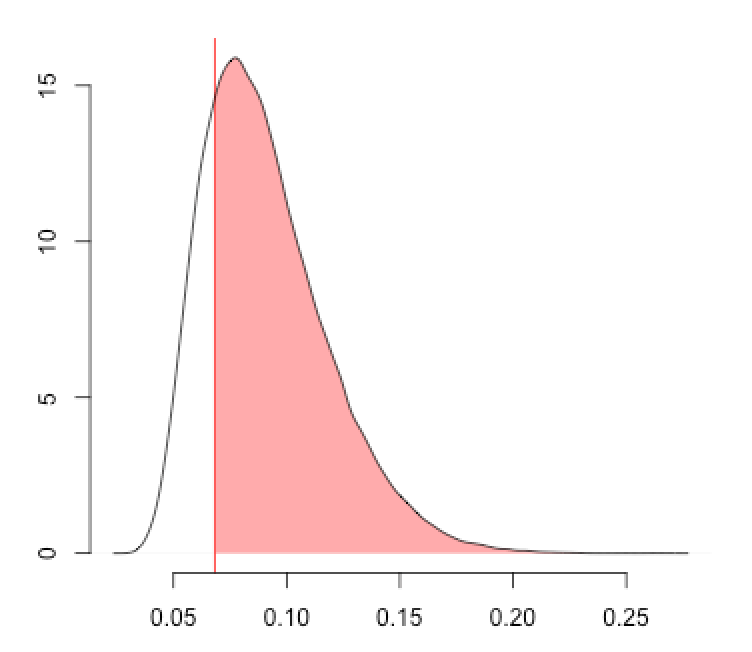# Monte Carlo techniques to create counterfactuals

October 11, 2018
By

Want to share your content on R-bloggers? click here if you have a blog, or here if you don't.

In the previous STT5100 course, last week, we’ve seen how to use monte carlo simulations. The idea is that we do observe in statistics a sample $\{y_1,\cdots,y_n\}$, and more generally, in econometrics $\{(y_1,\mathbf{x}_1),\cdots,(y_n,\mathbf{x}_n)\}$. But let’s get back to statistics (without covariates) to illustrate. We assume that observations $y_i$ are realizations of an underlying random variable $Y_i$. We assume that $Y_i$ are i.id. random variables, with (unkown) distribution $F_{\theta}$. Consider here some estimator $\widehat{\theta}$ – which is just a function of our sample $\widehat{\theta}=h(y_1,\cdots,y_n)$. So $\widehat{\theta}$ is a real-valued number like. Then, in mathematical statistics, in order to derive properties of the estimator $\widehat{\theta}$, like a confidence interval, we must define $\widehat{\theta}=h(Y_1,\cdots,Y_n)$, so that now, $\widehat{\theta}$ is a real-valued random variable. What is puzzling for students, is that we use the same notation, and I have to agree, that’s not very clever. So now, $\widehat{\theta}$ is.

There are two strategies here. In classical statistics, we use probability theorem, to derive properties of $\widehat{\theta}$ (the random variable) : at least the first two moments, but if possible the distribution. An alternative is to go for computational statistics. We have only one sample, $\{y_1,\cdots,y_n\}$, and that’s a pity. But maybe we can create another one $\{y_1^{(1)},\cdots,y_n^{(1)}\}$, as realizations of $F_{\theta}$, and another one $\{y_1^{(2)},\cdots,y_n^{(2)}\}$, anoter one $\{y_1^{(3)},\cdots,y_n^{(3)}\}$, etc. From those counterfactuals, we can now get a collection of estimators, $\widehat{\theta}^{(1)}$,$\widehat{\theta}^{(2)}$, $\widehat{\theta}^{(3)}$, etc. Instead of using mathematical tricks to calculate $\mathbb{E}(\widehat{\theta})$, compute $$\frac{1}{k}\sum_{s=1}^k\widehat{\theta}^{(s)}$$That’s what we’ve seen last friday.

I did also mention briefly that looking at densities is lovely, but not very useful to assess goodness of fit, to test for normality, for instance. In this post, I just wanted to illustrate this point. And actually, creating counterfactuals can we a good way to see it. Consider here the height of male students,

 1 2 3 4  Davis=read.table( "http://socserv.socsci.mcmaster.ca/jfox/Books/Applied-Regression-2E/datasets/Davis.txt") Davis[12,c(2,3)]=Davis[12,c(3,2)] X=Davis$height[Davis$sex=="M"]

We can visualize its distribution (density and cumulative distribution)

 1 2 3 4 5 6 7 8 9 10  u=seq(155,205,by=.5) par(mfrow=c(1,2)) hist(X,col=rgb(0,0,1,.3)) lines(density(X),col="blue",lwd=2) lines(u,dnorm(u,178,6.5),col="black") Xs=sort(X) n=length(X) p=(1:n)/(n+1) plot(Xs,p,type="s",col="blue") lines(u,pnorm(u,178,6.5),col="black")Since it looks like a normal distribution, we can add the density a Gaussian distribution on the left, and the cdf on the right. Why not test it properly. To be a little bit more specific, I do not want to test if it’s a Gaussian distribution, but if it’s a $\mathcal{N}(178,6.5^2)$. In order to see if this distribution is relevant, one can use monte carlo simulations to create conterfactuals

 1 2 3 4 5 6 7 8 9 10  hist(X,col=rgb(0,0,1,.3)) lines(density(X),col="blue",lwd=2) Y=rnorm(n,178,6.5) hist(Y,col=rgb(1,0,0,.3)) lines(density(Y),col="red",lwd=2) Ys=sort(Y) plot(Xs,p,type="s",col="white",lwd=2,axes=FALSE,xlab="",ylab="",xlim=c(155,205)) polygon(c(Xs,rev(Ys)),c(p,rev(p)),col="yellow",border=NA) lines(Xs,p,type="s",col="blue",lwd=2) lines(Ys,p,type="s",col="red",lwd=2)We can see on the left that it is hard to assess normality from the density (histogram and also kernel based density estimator). One can hardly think of a valid distance, between two densities. But if we look at graph on the right, we can compare the empirical distribution cumulative distribution $\widehat{F}$ obtained from $\{y_1,\cdots,y_n\}$ (the blue curve), and some conterfactual, $\widehat{F}^{(s)}$ obtained from $\{y_1^{(s)},\cdots,y_n^{(s)}\}$ generated from $F_{\theta_0}$ – where $\theta_0$ is the value we want to test. As suggested above, we can compute the yellow area, as suggest in Cramer-von Mises test, or the Kolmogorov-Smirnov distance.

 1 2 3 4 5 6 7 8 9 10  d=rep(NA,1e5) for(s in 1:1e5){ d[s]=ks.test(rnorm(n,178,6.5),"pnorm",178,6.5)$statistic } ds=density(d) plot(ds,xlab="",ylab="") dks=ks.test(X,"pnorm",178,6.5)$statistic id=which(ds$x>dks) polygon(c(ds$x[id],rev(ds$x[id])),c(ds$y[id],rep(0,length(id))),col=rgb(1,0,0,.4),border=NA) abline(v=dks,col="red")If we draw 10,000 counterfactual samples, we can visualize the distribution (here the density) of the distance used a test statistic $\widehat{d}^{(1)}$, $\widehat{d}^{(2)}$, etc, and compare it with the one observe on our sample $\widehat{d}$. The proportion of samples where the test-statistics exceeded the one observed

 1 2  mean(d>dks)  0.78248

is the computational version of the $p$-value

 1 2 3 4 5 6 7  ks.test(X,"pnorm",178,6.5)   One-sample Kolmogorov-Smirnov test   data: X D = 0.068182, p-value = 0.8079 alternative hypothesis: two-sided

I thought about all that a couple of days ago, since I got invited for a panel discussion on “coding”, and why “coding” helped me as professor. And this is precisely why I like coding : in statistics, either manipulate abstract objects, like random variables, or you actually use some lines of code to create counterfactuals, and generate fake samples, to quantify uncertainty. The later is interesting, because it helps to visualize complex quantifies. I do not claim that maths is useless, but coding is really nice, as a starting point, to understand what we talk about (which can be very usefull when there is a lot of confusion on notations).

R-bloggers.com offers daily e-mail updates about R news and tutorials about learning R and many other topics. Click here if you're looking to post or find an R/data-science job.
Want to share your content on R-bloggers? click here if you have a blog, or here if you don't.